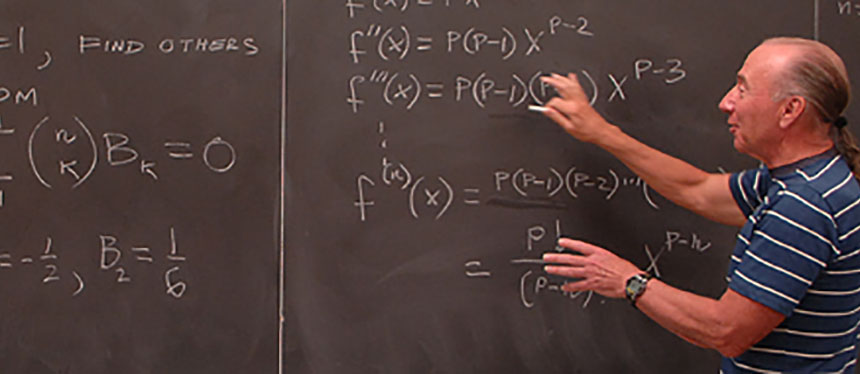# Minor in Applied Mathematics

•# Minor in Applied Mathematics

## Minor in Applied Mathematics

The applied mathematics minor consists of 21 semester hours and increases the mathematics major's ability to apply various fields of mathematics in the formulation, analysis and evaluation of problems in the physical, biological and social sciences. The minor provides the opportunity for students to participate in the dynamic character of modern mathematics and its uses.

Required courses: 18 s.h.

MATH 01.210 Linear Algebra
MATH 01.231 Ordinary Differential Equations
MATH 01.332 Numerical Analysis
STAT 02.360 Probability and Random Variables (formerly Probability and Statistics I)
MATH 03.400 Applications of Mathematics
CS 01.*** One course in Computer Science (Not 0701.100)

Elective courses (one): 3 s.h.

MATH 01.430 Complex Analysis
STAT 02.361 Mathematical Statistics (formerly Probability and Statistics II)
MATH 03.411 Deterministic Models in Operations Research
MATH 03.412 Stochastic Models in Operations Research
PHYS 02.315 Analytical Mechanics
PHYS 02.325 Mathematical Mechanics